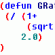# Understanding the 'if' function.## Recommended Posts```(defun gp:getPointInput	(/ StartPt EndPt HalfWidth)
(if (setq StartPt (getpoint "\nStart point of path: "))
(if (setq EndPt (getpoint StartPt "\nEndpoint of path: "))
(if (setq HalfWidth (getdist EndPt "\nhalf-width of path: "))
T
)
)
)
)```

I thought the syntax for the if function was:

(if (test expression)

(expression) (optional expression)

)

What is the purpose of using 'if' in the above code?

##### Share on other sitesThe intent may be clearer with better indentation:

```(defun gp:getPointInput ( / StartPt EndPt HalfWidth )
(if (setq StartPt (getpoint "\nStart point of path: "))
(if (setq EndPt (getpoint StartPt "\nEndpoint of path: "))
(if (setq HalfWidth (getdist EndPt "\nhalf-width of path: "))
T
)
)
)
)```

Here, the 'then' expressions for the first and second if statements are the second and third if statements respectively; though, the code is rather pointless since the 'then' expression for the final if statement is simply returning a boolean true value without using the collected points and distance values.

##### Share on other sitesThe intent may be clearer with better indentation:

```;;; Function gp:getPointInput will get path location and size
(defun gp:getPointInput	(/ StartPt EndPt HalfWidth)
(if (setq StartPt (getpoint "\nStart point of path: "))
(if	(setq EndPt (getpoint StartPt "\nEndpoint of path: "))
(if (setq HalfWidth (getdist EndPt "\nhalf-width of path: "))
(list StartPt EndPt HalfWidth)
)
)
)
)```

This is better? I've recently discovered the AutoLISP tutorial (that is where the code is from. I was asking about 'if' used as shown above because I have seen it so done before and wondered why. It didn't make sense when I was looking for something like:

(if (this statement is true)

(do this)

(else do this)

So essentially the 'test expression' for the first 'if' is "(getpoint "\nStart point of path: ")", the 'expression' to be evaluated if the 'test expression' returns a non-nil value is "(setq StartPt)", and the 'else' expression would be anything done by the user (eg. pressing 'Enter') that returns a nil value.

Am I correct?

##### Share on other sites```(defun gp:getPointInput ( / StartPt EndPt HalfWidth)
(if (setq StartPt (getpoint "\nStart point of path: "))           ;1
(if (setq EndPt (getpoint StartPt "\nEndpoint of path: "))       ;2
(if (setq HalfWidth (getdist EndPt "\nhalf-width of path: "))   ;3
(list StartPt EndPt HalfWidth)                                 ;4
)
)
)
)```

1. test if user picked a point or entered a set of coordinates; if succesful, go to next test.
2. test if user picked another point or entered a set of coordinates; if succesful, go to next test.
3. test if user picked a point (AutoCAD return measured distance between second point and current pick) or inputted a distance (numerical value).
4. if all three above inputs were valid (all tests were passed) the routine return a list with 1st and 2nd point, respectively the numerical value.

Mybe this formatting make more sense for you?

```(defun gp:getPointInput ( / StartPt EndPt HalfWidth)
(if (and (setq StartPt (getpoint "\nStart point of path: "))
(setq EndPt (getpoint StartPt "\nEndpoint of path: "))
(setq HalfWidth (getdist EndPt "\nhalf-width of path: ")))
(list StartPt EndPt HalfWidth)
)
)```

##### Share on other sitesI also annotated your code analisys:

So essentially the 'test expression' for the first 'if' is "(getpoint "\nStart point of path: ")", the 'expression' to be evaluated if the 'test expression' returns a non-nil value is "(setq StartPt)", and the 'else' expression would be anything done by the user (eg. pressing 'Enter') that returns a nil value.

So essentially the 'test expression' for the first 'if' is "(getpoint "\nStart point of path: ")" [true], the 'expression' to be evaluated if the 'test expression' returns a non-nil value is "(setq StartPt)", a new input test and the 'else' expression swould be anything done by the user (eg. pressing 'Enter') that returns a nil value. is not present into this piece of code; it does nothing if input is invalid or cancelled (more precisely the routine will return nil).

##### Share on other sitesMybe this formatting make more sense for you?

```(defun gp:getPointInput ( / StartPt EndPt HalfWidth)
(if (and (setq StartPt (getpoint "\nStart point of path: "))
(setq EndPt (getpoint StartPt "\nEndpoint of path: "))
(setq HalfWidth (getdist EndPt "\nhalf-width of path: ")))
(list StartPt EndPt HalfWidth)
)
)```

Yes, thanks.

So when you do multiple 'if' statements it is the same thing as a single 'if' statement with multiple 'and' test expressions.

##### Share on other sitesThere is a difference; using nested IF's will be able to define a specific action for each invalid input, while combining the tests with AND the action will be common. Please test in VLISP Console the two excerpts of code below:

```(if (setq var1st (getint "\nFirst number: "))
(if (setq var2nd (getint "\nSecond number: "))
)
)```

```(if (and (setq var1st (getint "\nFirst number: "))
(setq var2nd (getint "\nSecond number: ")))
)```

## Join the conversation

You can post now and register later. If you have an account, sign in now to post with your account.
Note: Your post will require moderator approval before it will be visible.Unfortunately, your content contains terms that we do not allow. Please edit your content to remove the highlighted words below.

×   Pasted as rich text.   Restore formatting

Only 75 emoji are allowed.